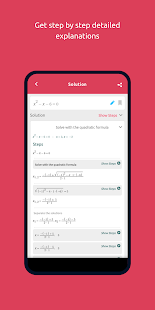# Symbolab – Math solver v9.6.1 [Pro] APK [Latest]

Feb 14, 2022Your private math tutor, solves any math problem with steps!

Your private math tutor, solves any math problem with steps! equations, integrals, derivatives, , limits and much more.
(Steps require a one-time in-app purchase)

Symbolab Math Solver app is composed of over a hundred Symbolab most powerful calculators:
Equation Calculator
Integral Calculator
Derivative Calculator
Limit calculator
Inequality Calculator
Trigonometry Calculator
Matrix Calculator
Functions Calculator
Series Calculator
ODE Calculator
Laplace Transform Calculator

Symbolab Math Solver solves any math problem including Pre- Algebra, Algebra, Pre-Calculus, Calculus, Trigonometry, Functions, Matrix, Vectors, Geometry and Statistics.

What’s New:
* Multiple choice problems support
* Enhancements and bug fixes

★ PRO / Paid features Unlocked
★ Unwanted Permissions + Activities removed
★ Unwanted Services + Receivers and Providers removed
★ Ads Activities + Services and Providers removed
★ AOSP compatible
★ Debug code removed
★ Original package signature changed
➡ Languages: Full Multi Languages
➡ CPU architectures: arm64-v8a, armeabi-v7a, x86, x86_64
➡ Screen DPIs: 120dpi, 160dpi, 240dpi, 320dpi, 480dpi, 640dpi

Screenshots

••••Symbolab – Math solver v9.6.1 [Pro Mod] APK / Mirror

Older Version

Symbolab – Math solver v9.4.0 [Pro Mod] APK / Mirror

Symbolab – Math solver v9.3.1 [Pro Mod] APK / Mirror